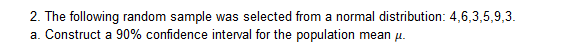# 2. The following random sample was selected from a normal distribution: 4,6,3,5,9,3a. Construct a 90% confidence interval for the population mean μ

Question
3 viewshelp_outlineImage Transcriptionclose2. The following random sample was selected from a normal distribution: 4,6,3,5,9,3 a. Construct a 90% confidence interval for the population mean μ fullscreen
check_circle

Step 1

In this question, we have to find the 90% confidence interval, with given data.

Sample Data is given below 4, 6, 3, 5, 9, and 3.

Step 2

Here we calculated the statistics that is useful to calculate the confidence interval. The required statistics are Mean and Standard Deviation. Calculations for mean and standard deviation have shown on whiteboard.

Step 3

Here, I have calculated the confidence interval and...

### Want to see the full answer?

See Solution

#### Want to see this answer and more?

Solutions are written by subject experts who are available 24/7. Questions are typically answered within 1 hour.*

See Solution
*Response times may vary by subject and question.
Tagged in

### Hypothesis Testing